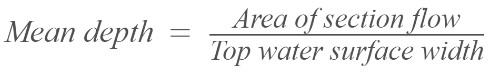# Mean Depth Calculator

To calculate mean depth, enter area & water surface width in required boxes and hit the calculate button using the mean depth calculator

Formula hm = AT

Give Us Feedback

Mean depth calculator is used to measure the mean depth, top water surface width, and area of section flow. It takes the numerical values of two terms to find the third term.

## What is mean depth?

The average water depth in a stream channel calculated by dividing the area of cross-section by the water surface width is known as the mean depth.

## Formulas of mean depth

• ### The formula used to calculate the mean depth is:hm = A/T

• ### The formula to find the area of section flow is:

Area of section flow = mean depth * top water surface width

A = hm * T

• ### The formula to compute the top water surface width is:T = A/hm

## How to find the mean depth?

Below is a solved example of the mean depth.

Example

Compute the mean depth, if the top water surface width is 50m and the cross-sectional area is 35m2

Solution

Step 1: Write the given data.

Mean depth = hm =?

Top water surface width = T = 50m

Cross-sectional area = A = 35m2

Step 2: Write the general formula of the mean depth.

Mean depth = area of section flow/top water surface width

hm = A/T

Step 3: Substitute the given data in the formula.

Mean depth = hm = 35m2/50m

Mean depth = hm = 7m/10

Mean depth = hm = 0.7m

### Math Tools